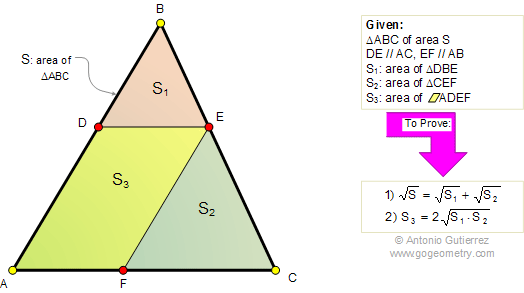# Online Geometry Problem 470. Triangle, Parallel, Parallelogram, Area, Similarity

The figure below shows a triangle ABC of area S, DE parallel to AC and EF parallel to AB. If S1, S2, and S3 are the areas of triangle DBE, triangle CEF, and parallelogram ADEF respectively, prove that (1), (2).Home | Geometry | Search | Problems | All Problems | 461-470 | Area of a Parallelogram | Email | Solution / comment | By Antonio Gutierrez